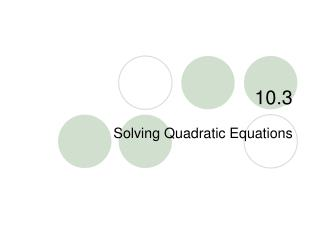DownloadDownload Presentation10.3

# 10.3

Download Presentation## 10.3

- - - - - - - - - - - - - - - - - - - - - - - - - - - E N D - - - - - - - - - - - - - - - - - - - - - - - - - - -
##### Presentation Transcript

2. 10.3 – Solving Quadratic Eq. • Goals / “I can…” • Solve quadratic equations by graphing • Solve quadratic equations using square roots

3. 10.3 – Solving Quadratic Eq. • When you look at a parabola, there are many important things to consider. • Vertex • Line of symmetry • y-intercept

4. 10.3 – Solving Quadratic Eq. • But one of the MOST IMPORTANT parts of the graph is where it crosses the x-axis or the x-intercept.

5. 10.3 – Solving Quadratic Eq. • To find the x-intercept, we use the quadratic equation. ax + bx + c = 0 • When you solve this equation, you will see 0, 1, or 2 solutions to the equations. What do I mean by this? 2

6. y x 10.3 – Solving Quadratic Eq. “0” solutions

7. y x 10.3 – Solving Quadratic Eq. “1” solutions

8. y x 10.3 – Solving Quadratic Eq. “2” solutions

9. 10.3 – Solving Quadratic Eq. • The x-intercept is also called the root OR zeros. To find the zeros we can graph or solve the equations.

10. 10.3 – Solving Quadratic Eq. y roots x O

11. 1 Solvex2 = 8 algebraically. 2 1 x2 = 8 2 CHECK 10.3 – Solving Quadratic Eq. Check your solution graphically. SOLUTION Write original equation. Multiply each side by 2. x2 = 16 Find the square root of each side. x =  4 Check these solutions using a graph.

12. 1 1 x2 = 8 2 1 x2– 8 = 0 2 CHECK Write the related function y =ax2 + bx + c. 2 1 y = x2– 8 2 10.3 – Solving Quadratic Eq. Check these solutions using a graph. Write the equation in the form ax 2 + bx +c= 0 Rewrite original equation. Subtract 8 from both sides.

13. 2 3 –4,0 4,0 1 y = x2– 8 2 1 y = Sketch graph of x2– 8. 2 CHECK 10.3 – Solving Quadratic Eq. Check these solutions using a graph. Write the related function y =ax2 + bx + c. The x-intercepts are  4, which agrees with the algebraic solution.

14. Solvex2 – x = 2 graphically. 1 2 Write the related function y =ax2 + bx + c. 10.3 – Solving Quadratic Eq. Check your solution algebraically. SOLUTION Write the equation in the form ax2 + bx + c = 0 x2 – x =2 Write original equation. Subtract 2 from each side. x2 – x – 2 = 0 y = x2 – x – 2

15. Write the related function y = ax2 + bx + c. 2 3 – 1,0 2,0 10.3 – Solving Quadratic Eq. y = x2 – x – 2 Sketch the graph of the function y = x2 – x – 2 From the graph, the x-interceptsappear to be x = –1 and x = 2

16. – 1,0 2,0 CHECK ? ? 22– 2 = 2 (–1)2– (–1) 2 = 10.3 – Solving Quadratic Eq. From the graph, the x-interceptsappear to be x = –1 and x =2 You can check this by substitution. Check x = 2: Check x = –1: x2– x = 2 x2– x = 2 4 – 2 = 2 1 + 1 = 2

17. 10.3 – Solving Quadratic Eq. • We can also find the zeroes by using a special function on our calculators. • So……..synchronize your calculators!!!

18. 10.3 – Solving Quadratic Eq. • graph x – 1 = 0 2x + 4 = 0 2 2

19. 10.3 – Solving Quadratic Eq. • Solve: 3x + 12 = 12 2

20. 10.3 – Solving Quadratic Eq. • Solve: x – 25 = 0 2